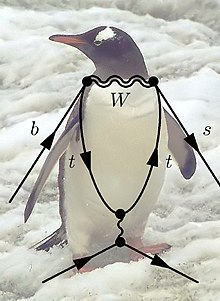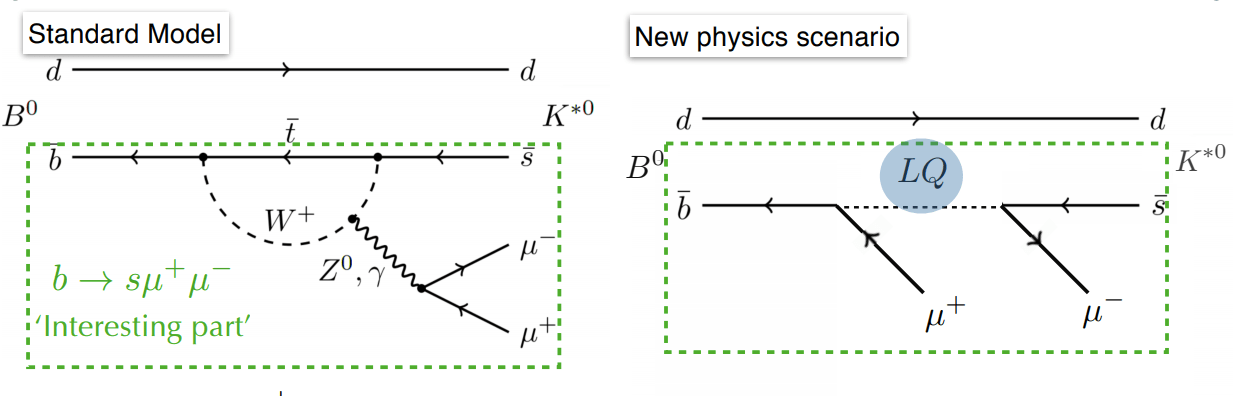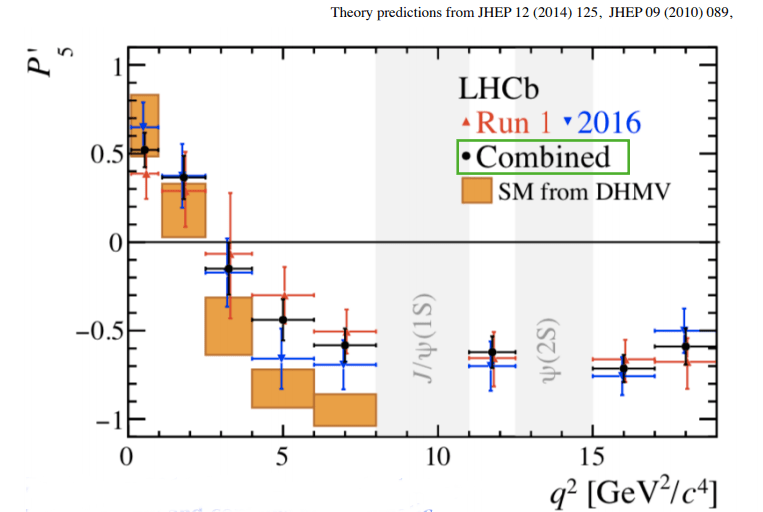## LHCb’s Flavor Mystery Deepens

Title: Measurement of CP -averaged observables in the B0→ K∗0µ+µ− decay

Authors: LHCb Collaboration

Refference: https://arxiv.org/abs/2003.04831

In the Standard Model, matter is organized in 3 generations; 3 copies of the same family of particles but with sequentially heavier masses. Though the Standard Model can successfully describe this structure, it offers no insight into why nature should be this way. Many believe that a more fundamental theory of nature would better explain where this structure comes from. A natural way to look for clues to this deeper origin is to check whether these different ‘flavors’ of particles really behave in exactly the same ways, or if there are subtle differences that may hint at their origin.

The LHCb experiment is designed to probe these types of questions. And in recent years, they have seen a series of anomalies, tensions between data and Standard Model predictions, that may be indicating the presence of new particles which talk to the different generations. In the Standard Model, the different generations can only interact with each other through the W boson, which means that quarks with the same charge can only interact through more complicated processes like those described by ‘penguin diagrams’.The so called ‘penguin diagrams’ describe how rare decays like bottom quark → strange quark can happen in the Standard Model. The name comes from both their shape and a famous bar bet. Who says physicists don’t have a sense of humor?

These interactions typically have quite small rates in the Standard Model, meaning that the rate of these processes can be quite sensitive to new particles, even if they are very heavy or interact very weakly with the SM ones. This means that studying these sort of flavor decays is a promising avenue to search for new physics.

In a press conference last month, LHCb unveiled a new measurement of the angular distribution of the rare B0→K*0μ+μ– decay. The interesting part of this process involves a b → s transition (a bottom quark decaying into a strange quark), where number of anomalies have been seen in recent years.Feynman diagrams of the decay being studied. A B meson (composed of a bottom and a down quark) decays into a Kaon (composed of a strange quark and a down quark) and a pair of muons. Because this decay is very rare in the Standard Mode (left diagram) it could be a good place to look for the effects of new particles (right diagram). Diagrams taken from here

Rather just measuring the total rate of this decay, this analysis focuses on measuring the angular distribution of the decay products. They also perform this mesaurement in different bins of ‘q^2’, the dimuon pair’s invariant mass. These choices allow the measurement to be less sensitive to uncertainties in the Standard Model prediction due to difficult to compute hadronic effects. This also allows the possibility of better characterizing the nature of whatever particle may be causing a deviation.

The kinematics of decay are fully described by 3 angles between the final state particles and q^2. Based on knowing the spins and polarizations of each of the particles, they can fully describe the angular distributions in terms of 8 parameters. They also have to account for the angular distribution of background events, and distortions of the true angular distribution that are caused by the detector. Once all such effects are accounted for, they are able to fit the full angular distribution in each q^2 bin to extract the angular coefficients in that bin.

This measurement is an update to their 2015 result, now with twice as much data. The previous result saw an intriguing tension with the SM at the level of roughly 3 standard deviations. The new result agrees well with the previous one, and mildly increases the tension to the level of 3.4 standard deviations.LHCb’s measurement of P’5, an observable describing one part of the angular distribution of the decay. The orange boxes show the SM prediction of this value and the red, blue and black point shows LHCb’s most recent measurement (a combination of its ‘Run 1’ measurement and the more recent 2016 data). The grey regions are excluded from the measurement because they have large backgrounds from the decays of other mesons.

This latest result is even more interesting given that LHCb has seen an anomaly in another measurement (the R_k anomaly) involving the same b → s transition. This had led some to speculate that both effects could be caused by a single new particle. The most popular idea is a so-called ‘leptoquark’ that only interacts with some of the flavors.

LHCb is already hard at work on updating this measurement with more recent data from 2017 and 2018, which should once again double the number of events. Updates to the R_k measurement with new data are also hotly anticipated. The Belle II experiment has also recent started taking data and should be able to perform similar measurements. So we will have to wait and see if this anomaly is just a statistical fluke, or our first window into physics beyond the Standard Model!

Symmetry Magazine “The mystery of particle generations”

Cern Courier “Anomalies persist in flavour-changing B decays”

Lecture Notes “Introduction to Flavor Physcis”

## Muon to electron conversion

Presenting: Section 3.2 of “Charged Lepton Flavor Violation: An Experimenter’s Guide”
Authors: R. Bernstein, P. Cooper
Reference1307.5787 (Phys. Rept. 532 (2013) 27)

Not all searches for new physics involve colliding protons at the the highest human-made energies. An alternate approach is to look for deviations in ultra-rare events at low energies. These deviations may be the quantum footprints of new, much heavier particles. In this bite, we’ll focus on the decay of a muon to an electron in the presence of a heavy atom.

The muon is a heavy version of the electron.There  are a few properties that make muons nice systems for precision measurements:

1. They’re easy to produce. When you smash protons into a dense target, like tungsten, you get lots of light hadrons—among them, the charged pions. These charged pions decay into muons, which one can then collect by bending their trajectories with magnetic fields. (Puzzle: why don’t pions decay into electrons? Answer below.)
2. They can replace electrons in atoms.  If you point this beam of muons into a target, then some of the muons will replace electrons in the target’s atoms. This is very nice because these “muonic atoms” are described by non-relativistic quantum mechanics with the electron mass replaced with ~100 MeV. (Muonic hydrogen was previous mentioned in this bite on the proton radius problem.)
3. They decay, and the decay products always include an electron that can be detected.  In vacuum it will decay into an electron and two neutrinos through the weak force, analogous to beta decay.
4. These decays are sensitive to virtual effects. You don’t need to directly create a new particle in order to see its effects. Potential new particles are constrained to be very heavy to explain their non-observation at the LHC. However, even these heavy particles can leave an  imprint on muon decay through ‘virtual effects’ according (roughly) to the Heisenberg uncertainty principle: you can quantum mechanically violate energy conservation, but only for very short times.Reach of muon conversion experiments from 1303.4097. The y axis is the energy scale that can be probed and the x axis parameterizes different ways that lepton flavor violation can appear in a theory.

One should be surprised that muon conversion is even possible. The process$\mu \to e$ cannot occur in vacuum because it cannot simultaneously conserve energy and momentum. (Puzzle: why is this true? Answer below.) However, this process is allowed in the presence of a heavy nucleus that can absorb the additional momentum, as shown in the comic at the top of this post.

Muon  conversion experiments exploit this by forming muonic atoms in the 1state and waiting for the muon to convert into an electron which can then be detected. The upside is that all electrons from conversion have a fixed energy because they all come from the same initial state: 1s muonic aluminum at rest in the lab frame. This is in contrast with more common muon decay modes which involve two neutrinos and an electron; because this is a multibody final state, there is a smooth distribution of electron energies. This feature allows physicists to distinguish between the$\mu \to e$ conversion versus the more frequent muon decay$\mu \to e \nu_\mu \bar \nu_e$ in orbit or muon capture by the nucleus (similar to electron capture).

The Standard Model prediction for this rate is miniscule—it’s weighted by powers of the neutrino to the W boson mass ratio  (Puzzle: how does one see this? Answer below.). In fact, the current experimental bound on muon conversion comes from the Sindrum II experiment  looking at muonic gold which constrains the relative rate of muon conversion to muon capture by the gold nucleus to be less than$7 \times 10^{-13}$. This, in turn, constrains models of new physics that predict some level of charged lepton flavor violation—that is, processes that change the flavor of a charged lepton, say going from muons to electrons.

The plot on the right shows the energy scales that are indirectly probed by upcoming muonic aluminum experiments: the Mu2e experiment at Fermilab and the COMET experiment at J-PARC. The blue lines show bounds from another rare muon decay: muons decaying into an electron and photon. The black solid lines show the reach for muon conversion in muonic aluminum. The dashed lines correspond to different experimental sensitivities (capture rates for conversion, branching ratios for decay with a photon). Note that the energy scales probed can reach 1-10 PeV—that’s 1000-10,000 TeV—much higher than the energy scales direclty probed by the LHC! In this way, flavor experiments and high energy experiments are complimentary searches for new physics.

These “next generation” muon conversion experiments are currently under construction and promise to push the intensity frontier in conjunction with the LHC’s energy frontier.

### Solutions to exercises:

1. Why do pions decay into muons and not electrons? [Note: this requires some background in undergraduate-level particle physics.] One might expect that if a charged pion can decay into a muon and a neutrino, then it should also go into an electron and a neutrino. In fact, the latter should dominate since there’s much more phase space. However, the matrix element requires a virtual W boson exchange and thus depends on an [axial] vector current. The only vector available from the pion system is its 4-momentum. By momentum conservation this is $p_\pi = p_\mu + p_\nu$. The lepton momenta then contract with Dirac matrices on the leptonic current to give a dominant piece proportional to the lepton mass. Thus the amplitude for charged pion decay into a muon is much larger than the amplitude for decay into an electron.
2. Why can’t a muon decay into an electron in vacuum? The process$\mu \to e$ cannot simultaneously conserve energy and momentum. This is simplest to see in the reference frame where the muon is at rest. Momentum conservation requires the electron to also be at rest. However, a particle has rest energy equal to its mass, but now there’s now way a muon at rest can pass on all of its energy to an electron at rest.
3. Why is muon conversion in the Standard Model suppressed by the ration of the neutrino to W masses? This can be seen by drawing the Feynman diagram (fig below from 1401.6077). Flavor violation in the Standard Model requires a W boson. Because the W is much heavier than the muon, this must be virtual and appear only as an internal leg. Further, W‘s couple charged leptons to neutrinos, so there must also be a virtual neutrino. The evaluation of this diagram into an amplitude gives factors of the neutrino mass in the numerator (required for the fermion chirality flip) and the W mass in the denominator. For some details, see this post.